Saturday, August 15, 2020
Home > CBSE Class 12 > NCERT Solutions for Class 12 Maths Continuity and Differentiability Exercise 5.5

# NCERT Solutions for Class 12 Maths Continuity and Differentiability Exercise 5.5# NCERT Solutions for Class 12 Maths Continuity and Differentiability

Hi Students, Welcome to Amans Maths Blogs (AMB). In this post, you will get the NCERT Solutions for Class 12 Maths Continuity and Differentiability Exercise 5.5.

CBSE Class 12th is an important school class in your life as you take some serious decision about your career. And out of all subjects, Maths is an important and core subjects. So CBSE NCERT Solutions for Class 12th Maths is major role in your exam preparation as it has detailed chapter wise solutions for all exercise. This NCERT Solutions can be downloaded in PDF file. The downloading link is given at last.

NCERT Solutions for Class 12 Maths are not only the solutions of Maths exercise but it builds your foundation of other important subjects. Getting knowledge of depth concept of CBSE Class 12th Maths like Algebra, Calculus, Trigonometry, Coordinate Geometry help you to understand the concept of Physics and Physical Chemistry.

As we know that all the schools affiliated from CBSE follow the NCERT books for all subjects. You can check the CBSE NCERT Syllabus. Thus, NCERT Solutions helps the students to solve the exercise questions as given in NCERT Books.

## NCERT Solutions for Class 12 Maths Continuity and Differentiability Exercise 5.5

Differentiate the functions given in Exercises 1 to 11 w.r.t. x.

NCERT Solutions for Class 12 Maths Continuity and Differentiability Exercise 5.5: Ques No 1.

cos x . cos 2x . cos 3x

NCERT Solutions: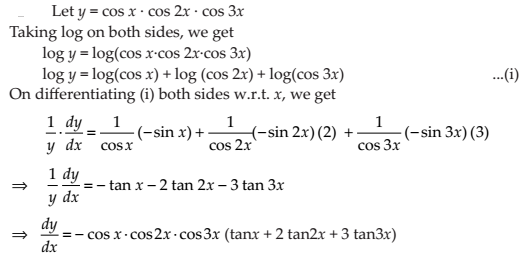NCERT Solutions for Class 12 Maths Continuity and Differentiability Exercise 5.5: Ques No 2.NCERT Solutions: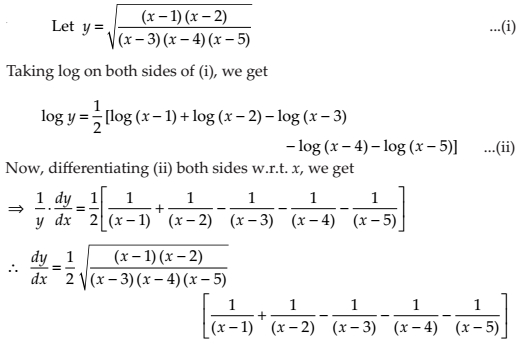NCERT Solutions for Class 12 Maths Continuity and Differentiability Exercise 5.5: Ques No 3.

(log x)cos x

NCERT Solutions:NCERT Solutions for Class 12 Maths Continuity and Differentiability Exercise 5.5: Ques No 4.

xx – 2sin x

NCERT Solutions:NCERT Solutions for Class 12 Maths Continuity and Differentiability Exercise 5.5: Ques No 5.

(x + 3)2 . (x + 4)3 . (x + 5)4

NCERT Solutions: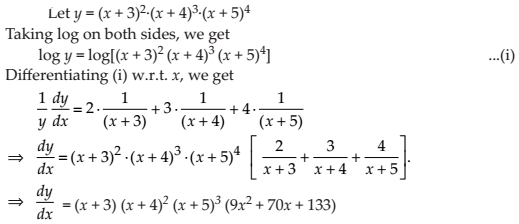NCERT Solutions for Class 12 Maths Continuity and Differentiability Exercise 5.5: Ques No 6.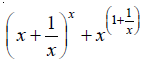NCERT Solutions: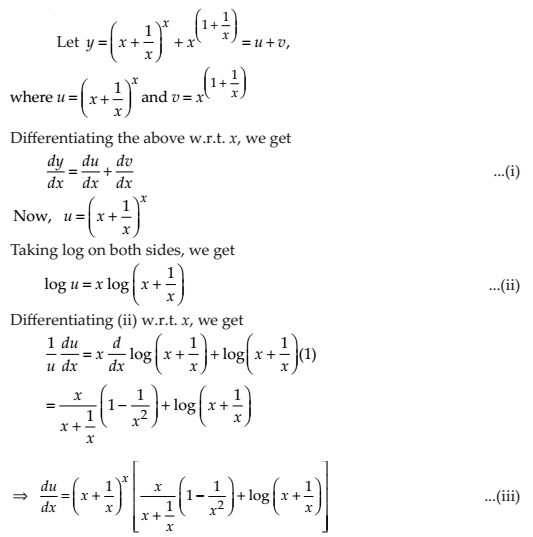NCERT Solutions for Class 12 Maths Continuity and Differentiability Exercise 5.5: Ques No 7.

(log x)x + xlog x

NCERT Solutions: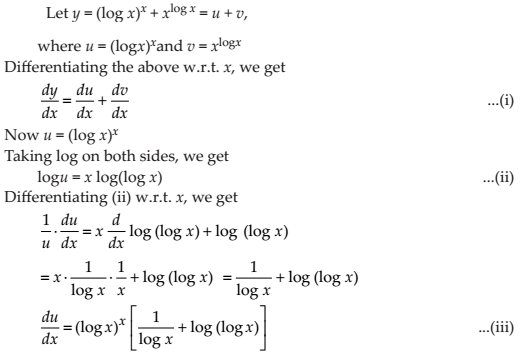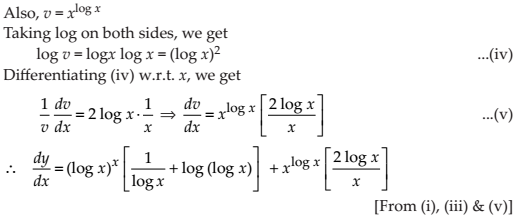NCERT Solutions for Class 12 Maths Continuity and Differentiability Exercise 5.5: Ques No 8.NCERT Solutions: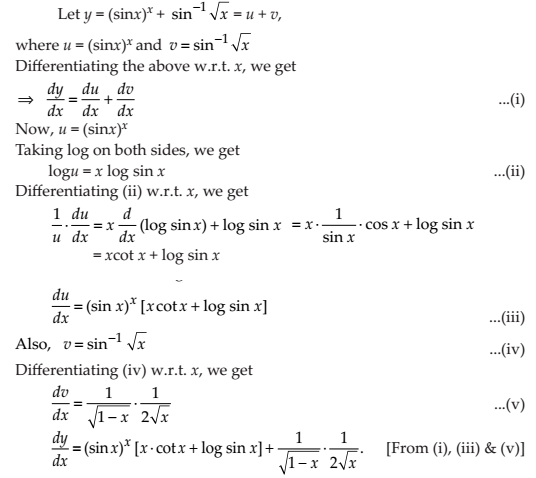NCERT Solutions for Class 12 Maths Continuity and Differentiability Exercise 5.5: Ques No 9.

xsin x + (sin x)cos x

NCERT Solutions: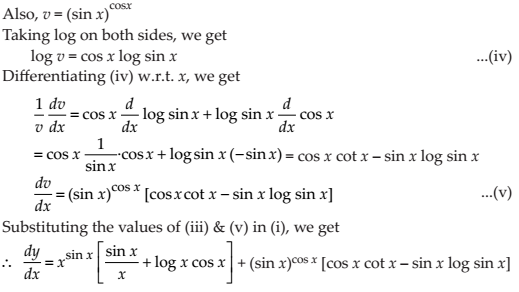NCERT Solutions for Class 12 Maths Continuity and Differentiability Exercise 5.5: Ques No 10.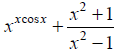NCERT Solutions:NCERT Solutions for Class 12 Maths Continuity and Differentiability Exercise 5.5: Ques No 11.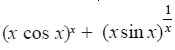NCERT Solutions: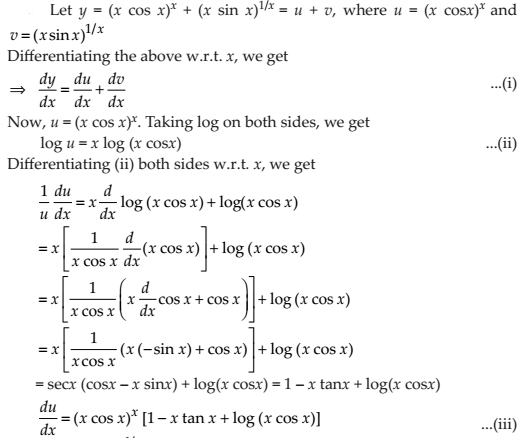Find dy/dx of the functions given in Exercises 12 to 15.

NCERT Solutions for Class 12 Maths Continuity and Differentiability Exercise 5.5: Ques No 12.

xy + yx = 1

NCERT Solutions: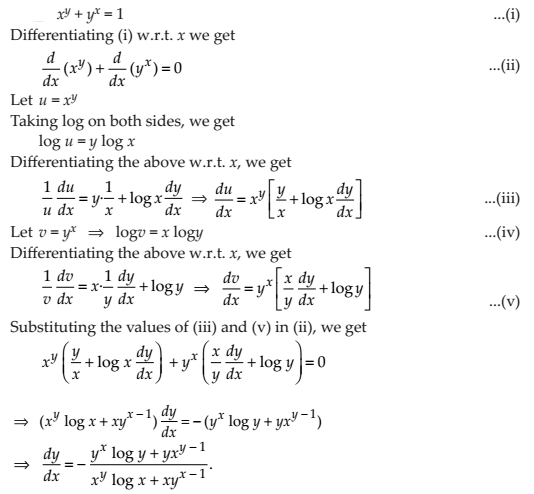NCERT Solutions for Class 12 Maths Continuity and Differentiability Exercise 5.5: Ques No 13.

yx = xy

NCERT Solutions: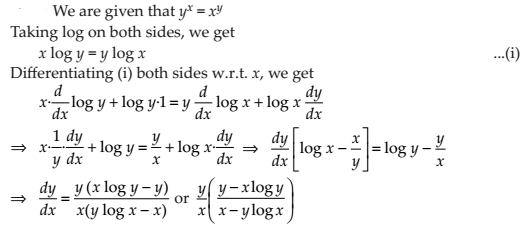NCERT Solutions for Class 12 Maths Continuity and Differentiability Exercise 5.5: Ques No 14.

(cos x)y = (cos y)x

NCERT Solutions: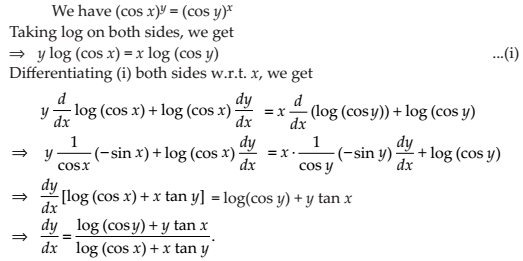NCERT Solutions for Class 12 Maths Continuity and Differentiability Exercise 5.5: Ques No 15.

xy = e(x – y)

NCERT Solutions:NCERT Solutions for Class 12 Maths Continuity and Differentiability Exercise 5.5: Ques No 16.

Find the derivative of the function given by f (x) = (1 + x)(1 + x2)(1 + x4)(1 + x8) and hence find f ′(1).

NCERT Solutions:NCERT Solutions for Class 12 Maths Continuity and Differentiability Exercise 5.5: Ques No 17.

Differentiate (x2 – 5x + 8)(x3 + 7x + 9) in three ways mentioned below:

(i) by using product rule

(ii) by expanding the product to obtain a single polynomial.

(iii) by logarithmic differentiation

NCERT Solutions: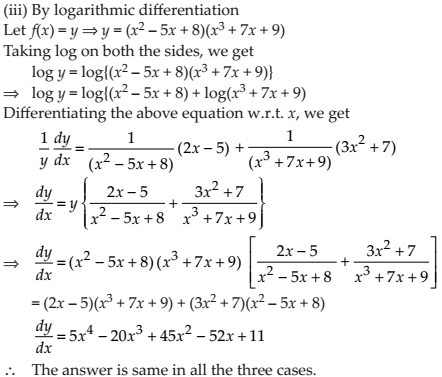NCERT Solutions for Class 12 Maths Continuity and Differentiability Exercise 5.5: Ques No 18.

If u, v and w are functions of x, then show that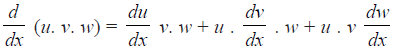in two ways – first by repeated application of product rule, second by logarithmic differentiation.

NCERT Solutions:error: Content is protected !!